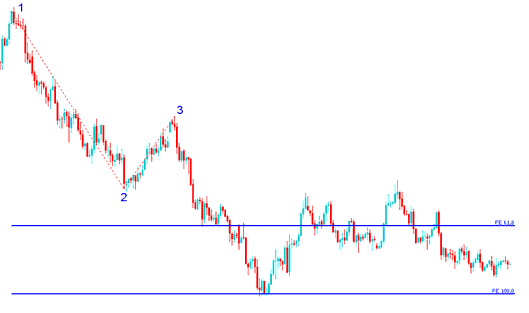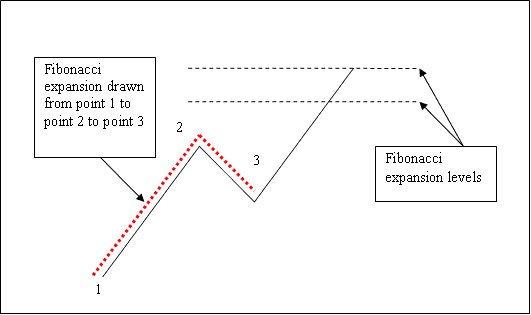# How to Draw Indices Trading Fibonacci Extension Indicator Tool on Downwards Indices Trend

## How Do I Draw Indices Trading Fibonacci Extension for Indices Trading Downtrend?

We draw the Indices Trading Fibonacci Extension indicator tool from chart point 1 to chart point 2 to chart point 3 as shown on the stock indexes trading example explained and illustrated below. Remember we always draw this Indices Trading Fibonacci Extension tool in the direction of the indices trend. In the Indices Trading Fibonacci Extension example explained and illustrated below, can you figure what direction we have drawn it? That is right - downward direction.

As a indices trader you would also have used Indices Trading Fibonacci Extension level 100.0%, just where the stock indexes price reached as shown on the Indices Trading Fibonacci Extension example explained and illustrated below. That would have been a good take profit area.How to Draw Indices Trading Fibonacci Extension on Down Indices Trend

From the Indices Trading Fibonacci Extension indicator tool example above, after drawing this Indices Trading Fibonacci Extension indicator tool there are two levels that are used to show the profit taking levels, these two Indices Trading Fibonacci Extension levels are drawn as horizontal lines across the stock indices chart marked as 61.8% Indices Trading Fibonacci Extension Level and 100% Indices Trading Fibonacci Extension Level.

## Explanation of How to Draw Indices Trading Fibonacci Extension Levels on Stock Indexes Charts

We use Indices Trading Fibonacci Extensionlevels to estimate where the indices trend movement will reach. There are 2 commonly used Indices Trading Fibonacci Extension levels; 61.8% Indices Trading Fibonacci Extension Level and 100% Indices Trading Fibonacci Extension Levels, these are used for setting taking profit levels.

To draw Indices Trading Fibonacci Extension levels on the stock indices chart we wait until the stock indexes price retracement is complete and the stock indexes price starts to move in the original direction of the Indices trend. Where the stock indexes price retracement reaches is used as chart point 3. Indices Trading Fibonacci Extension Indicator tool is drawn using three chart points as shown on the stock indexes trading example explained and illustrated below of how to draw the Indices Trading Fibonacci Extension Indicator Tool.

The Indices Trading Fibonacci Extension example explained and illustrated below shows the 3 Chart Points where the Indices Trading Fibonacci Extension indicator is drawn, marked as Chart point 1, Chart point 2 and Chart point 3.

Chart point 1 is where the indices trend started, Chart point 2 is where the indices trend pulled back and retraced and Chart point 3 is where the stock indices retracement reached as shown on the Indices Trading Fibonacci Extension Indicator Tool example explained and illustrated below.How to Draw Indices Trading Fibonacci Extension Indicator Tool on Downwards Indices Trend

Please note where these Indices Trading Fibonacci Extension levels are drawn - Indices Trading Fibonacci Extension levels are drawn above the Fibonacci indicator, these are the chart points where a indices trader will set the take profit orders using these Indices Trading Fibonacci Extension Levels - 61.8% Indices Trading Fibonacci Extension Level and 100.0% Indices Trading Fibonacci Extension Level.